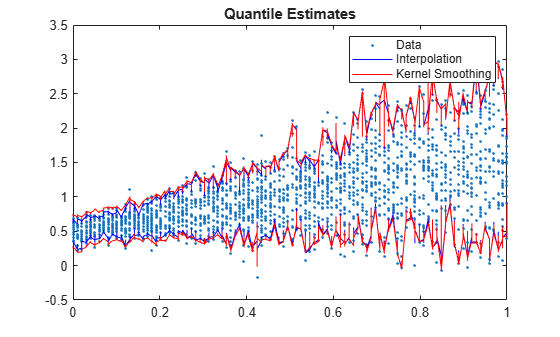Main Content

# Conditional Quantile Estimation Using Kernel Smoothing

This example shows how to estimate conditional quantiles of a response given predictor data using quantile random forest and by estimating the conditional distribution function of the response using kernel smoothing.

For quantile-estimation speed, `quantilePredict`, `oobQuantilePredict`, `quantileError`, and `oobQuantileError` use linear interpolation to predict quantiles in the conditional distribution of the response. However, you can obtain response weights, which comprise the distribution function, and then pass them to `ksdensity` to possibly gain accuracy at the cost of computation speed.

Generate 2000 observations from the model

`${y}_{t}=0.5+t+{\epsilon }_{t}.$`

$t$ is uniformly distributed between 0 and 1, and ${\epsilon }_{t}\sim N\left(0,{t}^{2}/2+0.01\right)$. Store the data in a table.

```n = 2000; rng('default'); % For reproducibility t = randsample(linspace(0,1,1e2),n,true)'; epsilon = randn(n,1).*sqrt(t.^2/2 + 0.01); y = 0.5 + t + epsilon; Tbl = table(t,y);```

Train an ensemble of bagged regression trees using the entire data set. Specify 200 weak learners and save the out-of-bag indices.

```rng('default'); % For reproducibility Mdl = TreeBagger(200,Tbl,'y','Method','regression',... 'OOBPrediction','on');```

`Mdl` is a `TreeBagger` ensemble.

Predict out-of-bag, conditional 0.05 and 0.95 quantiles (90% confidence intervals) for all training-sample observations using `oobQuantilePredict`, that is, by interpolation. Request response weights. Record the execution time.

```tau = [0.05 0.95]; tic [quantInterp,yw] = oobQuantilePredict(Mdl,'Quantile',tau); timeInterp = toc;```

`quantInterp` is a 94-by-2 matrix of predicted quantiles; rows correspond to the observations in `Mdl.X` and columns correspond to the quantile probabilities in `tau`. `yw` is a 94-by-94 sparse matrix of response weights; rows correspond to training-sample observations and columns correspond to the observations in `Mdl.X`. Response weights are independent of `tau`.

Predict out-of-bag, conditional 0.05 and 0.95 quantiles using kernel smoothing and record the execution time.

```n = numel(Tbl.y); quantKS = zeros(n,numel(tau)); % Preallocation tic for j = 1:n quantKS(j,:) = ksdensity(Tbl.y,tau,'Function','icdf','Weights',yw(:,j)); end timeKS = toc;```

`quantKS` is commensurate with `quantInterp`.

Evaluate the ratio of execution times between kernel smoothing estimation and interpolation.

`timeKS/timeInterp`
```ans = 4.3864 ```

It takes much more time to execute kernel smoothing than interpolation. This ratio is dependent on the memory of your machine, so your results will vary.

Plot the data with both sets of predicted quantiles.

```[sT,idx] = sort(t); figure; h1 = plot(t,y,'.'); hold on h2 = plot(sT,quantInterp(idx,:),'b'); h3 = plot(sT,quantKS(idx,:),'r'); legend([h1 h2(1) h3(1)],'Data','Interpolation','Kernel Smoothing'); title('Quantile Estimates') hold off```Both sets of estimated quantiles agree fairly well. However, the quantile intervals from interpolation appear slightly tighter for smaller values of `t` than the ones from kernel smoothing.

Download ebook